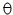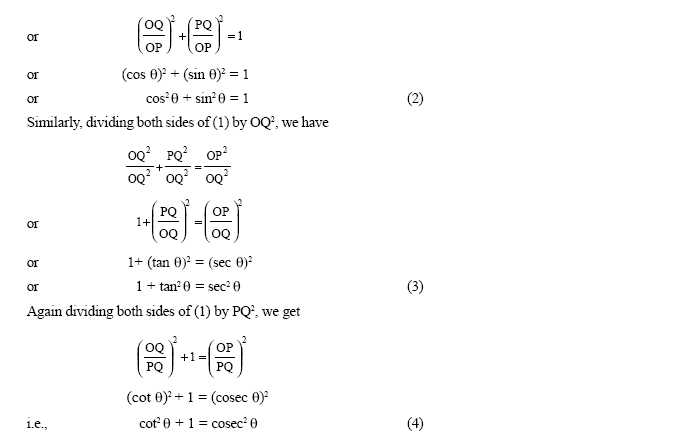Email us to get an instant 20% discount on highly effective K-12 Math & English kwizNET Programs!

#### Online Quiz (WorksheetABCD)

Questions Per Quiz = 2 4 6 8 10

### Geometry8.11 Trignometry Identities

 Consider any acute angle AOB. P is a point on the ray OB and PQ is perpendicular to OA.We have Sin= PQ/OP Cos= OQ/OP Tan= PQ/OQ Cosec= 1/Sin= OP/PQ Sec= 1/Cos= OP/OQ Cot= 1/tan= OQ/OP Important identities : sin2+ cos2= 1 1 + tan2= sec21 + cot2= cosec2Example: Show that cot+ tan= sec.coseccot+ tan= cos/sin+ sin/cos= cos2+sin2/sincos= 1/sincos= 1/sin. 1/cos= cosec.secDirections: Find the mid point of the segment joining two points and draw a graph for the problems.
 Q 1: Prove that tan x + cot x = sec x. cosec xAnswer: Q 2: Prove that (sec x + tan x -1)(sec x - tan x + 1) - 2 tan x = 0Answer: Q 3: If cos x + sin x = 21/2 cos x, prove that cos x - sin x = 21/2 sin x.Answer: Q 4: If sin x + cosec x = 2, prove htat sin2 + cosec 2 = 2.Answer: Q 5: Prove that (1+cot x - cosec x)(1 +tan x + sec x) = 2Answer: Q 6: Prove that (1+tanx)2 + (1-tanx)2 = 2 sec2x.Answer: Q 7: Prove that cos x/(sec x - tan x) = 1 + sin xAnswer: Q 8: Prove that sin y/(1+cosy) + (1+cos y)/sin y = 2 cosec yAnswer: Question 9: This question is available to subscribers only! Question 10: This question is available to subscribers only!